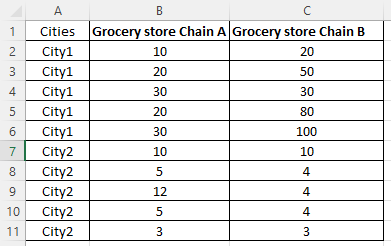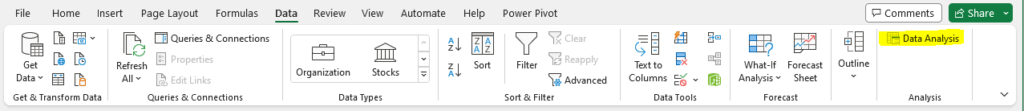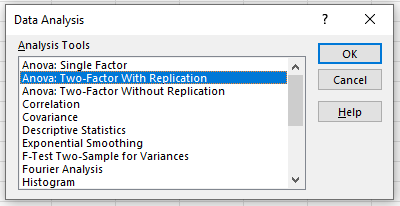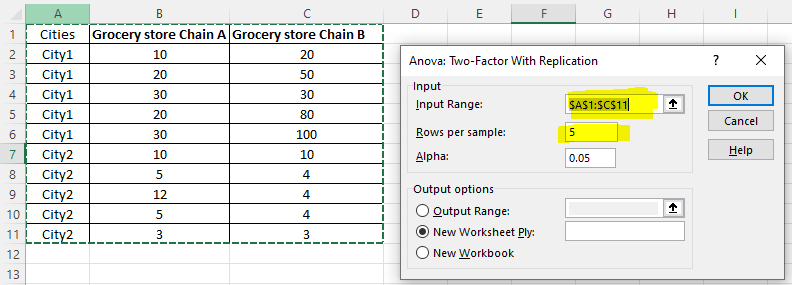# Two-Way ANOVA in Excel

This tutorial shows how you can do two-way ANOVA in Excel. Different from one-way ANOVA, two-way ANOVA has two independent variables. Note that, two-way ANOVA is also called two-factor ANOVA.

## Prepare data and hypothesis writing

Suppose Brand A sells its products in city1 and city2, each city with 2 grocery store chains (Grocery store Chain A and Grocery store Chain B). Each grocery store chain has 5 stores in each city.

You can download this Excel file of two-way ANOVA used in this tutorial via GitHub.Thus, two-way ANOVA can test the following hypotheses.

Null hypothesis: The sales difference between Grocery store Chain A and Grocery store Chain B does not differ between city1 and city2.
Alternative hypothesis: The sales difference between Grocery store Chain A and Grocery store Chain B does differ between city1 and city2.

## Steps of Two-way ANOVA in Excel

1. Click Data Analysis on the top menu.

If you can not find it, please check my another tutorial – How to fix: Data Analysis not Show up in Excel.2. Select “ANOVA: Two-Factor with Replication” and then click “OK.”3. Select A1 to C11 into “Input Range“. Remember to include the first row and first column.

Then, set “Rows per sample” to 5, since each chain has 5 stores in each city. Then, click “OK“.### Report findings of Two-way ANOVA

Based on the output shown below, you can report the two-way ANOVA Excel results as follows:

We tested two-way ANOVA and found that F(1,16) = 5.30, p = 0.035. Since the p-value = 0.035, which is smaller than 0.05, we reject the null hypothesis. We thus conclude that the sales difference between Grocery store Chain A and Grocery store Chain B does differ between city1 and city2.